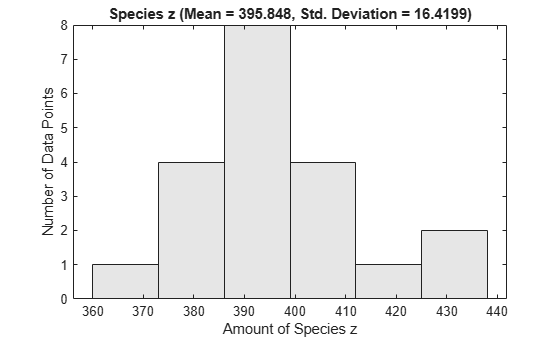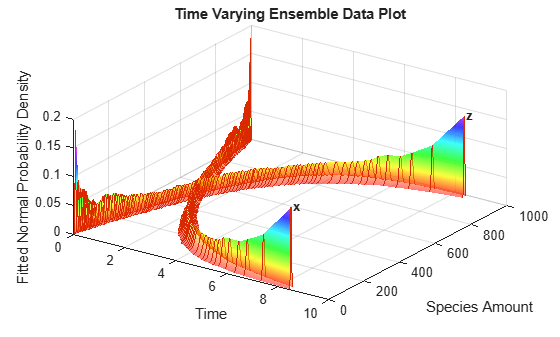# sbioensemblerun

Multiple stochastic ensemble runs of SimBiology model

## Syntax

```simdataObj = sbioensemblerun(modelObj, Numruns) simdataObj = sbioensemblerun(modelObj, Numruns, Interpolation) simdataObj = sbioensemblerun(modelObj, Numruns, configsetObj) simdataObj = sbioensemblerun(modelObj, Numruns, configsetObj, Interpolation) simdataObj = sbioensemblerun(modelObj, Numruns, variantObj) simdataObj = sbioensemblerun(modelObj, Numruns, variantObj, Interpolation) simdataObj = sbioensemblerun(modelObj, Numruns, configsetObj, variantObj) simdataObj = sbioensemblerun(modelObj, Numruns, configsetObj, variantObj, Interpolation) ```

## Arguments

 `simdataObj` An array of `SimData objects` containing simulation data generated by `sbioensemblerun`. All elements of `simdataObj` contain data for the same states in the same model. `modelObj` Model object to be simulated. `Numruns` Integer scalar representing the number of stochastic runs to make. `Interpolation` Character vector or string denoting the interpolation scheme to be used if data should be interpolated to get a consistent time vector. Valid values are `'linear'` (linear interpolation), `'zoh'` (zero-order hold), or `'off'` (no interpolation). Default is `'off'`. If interpolation is on, the data is interpolated to match the time vector with the smallest simulation stop time. `configsetObj` Specify the configuration set object to use in the ensemble simulation. For more information about configuration sets, see `Configset object`. `variantObj` Specify the variant object to apply to the model during the ensemble simulation. For more information about variant objects, see `Variant object`.

## Description

`simdataObj = sbioensemblerun(modelObj, Numruns)` performs a stochastic ensemble run of the SimBiology® model object (`modelObj`), and returns the results in `simdataObj`, an array of `SimData objects`. The active configset and the active variants are used during simulation and are saved in the output, SimData object (`simdataObj`).

`sbioensemblerun` uses the settings in the active configset on the model object (`modelObj`) to perform the repeated simulation runs. The `SolverType` property of the active configset must be set to one of the stochastic solvers: `'ssa'`, `'expltau'`, or `'impltau'`. `sbioensemblerun` generates an error if the `SolverType` property is set to any of the deterministic (ODE) solvers.

`simdataObj = sbioensemblerun(modelObj, Numruns, Interpolation)` performs a stochastic ensemble run of a model object (`modelObj`), and interpolates the results of the ensemble run onto a common time vector using the interpolation scheme (`Interpolation`).

`simdataObj = sbioensemblerun(modelObj, Numruns, configsetObj)` performs an ensemble run of a model object (`modelObj`), using the specified configuration set (`configsetObj`).

`simdataObj = sbioensemblerun(modelObj, Numruns, configsetObj, Interpolation)` performs an ensemble run of a model object (`modelObj`), using the specified configuration set (`configsetObj`), and interpolates the results of the ensemble run onto a common time vector using the interpolation scheme (`Interpolation`).

`simdataObj = sbioensemblerun(modelObj, Numruns, variantObj)` performs an ensemble run of a model object (`modelObj`), using the variant object or array of variant objects (`variantObj`).

`simdataObj = sbioensemblerun(modelObj, Numruns, variantObj, Interpolation)` performs an ensemble run of a model object (`modelObj`), using the variant object or array of variant objects (`variantObj`), and interpolates the results of the ensemble run onto a common time vector using the interpolation scheme (`Interpolation`).

`simdataObj = sbioensemblerun(modelObj, Numruns, configsetObj, variantObj)` performs an ensemble run of a model object (`modelObj`), using the configuration set (`configsetObj`), and the variant object or array of variant objects (`variantObj`). If the configuration set object (`configsetObj`) is empty, the active configset on the model is used for simulation. If the variant object (`variantObj`) is empty, then no variant (not even the active variants in the model) is used for the simulation.

`simdataObj = sbioensemblerun(modelObj, Numruns, configsetObj, variantObj, Interpolation)` performs an ensemble run of a model object (`modelObj`), using the configuration set (`configsetObj`), and the variant object or array of variant objects (`variantObj`), and interpolates the results of the ensemble run onto a common time vector using the interpolation scheme (`Interpolation`).

## Examples

collapse all

`sbioloadproject("radiodecay.sbproj");`

Change the solver of the active configuration set to be `ssa`. Also, adjust the `LogDecimation` property on the `SolverOptions` property of the configuration set.

```cs = getconfigset(m1,"active"); cs.SolverType = "ssa"; so = cs.SolverOptions; so.LogDecimation = 10;```

Perform an ensemble of 20 runs with no interpolation.

```ensembleData = sbioensemblerun(m1,20); sbioensembleplot(ensembleData, 'z', 1.0);```Create a 2-D distribution plot of the species z at time = 1.0.

`sbioensembleplot(ensembleData,"z",1.0); `Get ensemble statistics for all species using the default interpolation method.

`[T,M,V] = sbioensemblestats(ensembleData);`

Get ensemble statistics for a specific species using the default interpolation scheme.

`[T2,M2,V2] = sbioensemblestats(ensembleData,"z");`

Create a 3-D shaded plot of the species x and z.

`sbioensembleplot(ensembleData,["x","z"]);`## Version History

Introduced in R2006a